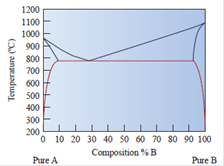Create an Account

Home / Questions / The copper-silver phase diagram is shown in Figure 11-30 Copper has a higher melting point...

The copper-silver phase diagram is shown in Figure 11-30 Copper has a higher melting point than silver. Refer to the silver-rich solid phase as gamma and the copper rich solid phase as delta

The copper-silver phase diagram is shown in Figure 11-30. Copper has a higher melting point than silver. Refer to the silver-rich solid phase as gamma (?) and the copper rich solid phase as delta (d). Denote the liquid as L.Figure 11-30A phase diagram for elements A and B

(a) For an overall composition of 60% B (40% A) at a temperature of 800°C, what are the compositions and amounts of the phases present?

(b) For an overall composition of 30% B (70% A) at a temperature of 1000°C, what are the compositions and amounts of the phases present?

(c) Draw a schematic diagram illustrating the final

May 20 2020 View more View LessSubscribe To Get Solution